• Shuffle
Toggle On
Toggle Off
• Alphabetize
Toggle On
Toggle Off
• Front First
Toggle On
Toggle Off
• Both Sides
Toggle On
Toggle Off
Toggle On
Toggle Off
Front

## Card Range To Study

throughPlay buttonPlay buttonProgress

1/22

Click to flip

Use LEFT and RIGHT arrow keys to navigate between flashcards;

Use UP and DOWN arrow keys to flip the card;

H to show hint;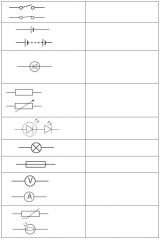Circuit symbols - What do these symbols mean?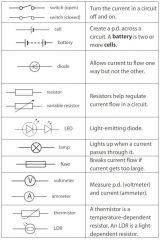What is Potential difference? Potential difference is a measure of the difference in energy between two parts of a circuit. The bigger the difference in energy, the bigger the potential difference. What is Current? Current is a measure of how much electric charge flows through a circuit. The more charge that flows, the bigger the current. What is Resistance? The wires and the other components in a circuit reduces the flow of charge through them. This is called resistance. What is the unit of resistance? The unit of resistance is the ohm, and it has the symbol Ω (an uppercase Greek letter omega). What is the unit of current? Current is measured in amperes. The symbol for ampere is A. What is the unit of Potential difference? Potential difference is measured in volts. The symbol for volts is V. What does Ohms Law state? Ohms (Ω) = volts (V) / amperes (A)The current flowing through a resistor at a constant temperature is directly proportional to the voltage across the resistor. So, if you double the voltage, the current also doubles. This is called Ohm's Law. A lamp has a resistance of 3 ohms. It takes a current of 0.6 A. What voltage does it work at? resistance = voltage / currentRearrange this to:voltage = current x resistanceSo the voltage in the lamp is 3 x 0.6 = 1.8 volts Explain what is meant by a series circuit.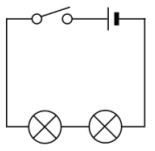Components that are connected one after another on the same loop of the circuit are connected in series. The current that flows in each component connected in series is the same. Explain what is meant by a parallel circuit.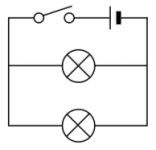In a parallel circuit, different components are connected on different branches of the wire. When components are connected in parallel, the current is shared between the components. Explain how to measure the current in a circuit.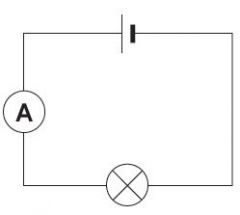A device called an ammeter is used to measure current. To measure the current flowing through a component in a circuit, you must connect the ammeter in series with it. Explain how to measure the voltage in a circuit.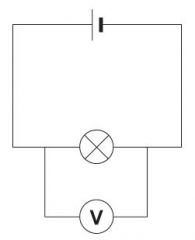Voltage is measured using a voltmeter. To measure the voltage across a component in a circuit, you must connect the voltmeter in parallel with it. What is a thermistor?What is its symbol?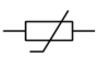Thermistors are devices which can alter their resistance with temperature: At low temperatures, the resistance of a thermistor is high, and little current can flow through them.At high temperatures, the resistance of a thermistor is low, and more current can flow through them. What is a LDR?What is its symbol?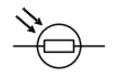LDRs (light-dependent resistors) are devices which can alter their resistance with light. In the dark and at low light levels, the resistance of an LDR is high, and little current can flow through it.In bright light, the resistance of an LDR is low, and more current can flow through it.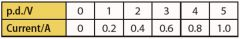Calculate the current through a 60 Ω resistor if a p.d. of 12 V is applied to it. Use the data in the table to draw a graph of p.d.and current for a resistor. I = V/R, so I =12/60 = 0.2 A. p.d. (V), x axis; current (A), y axis; line of best fit through all points. Describe the sequence of events in an electric oven controlled by a thermostat as it comes up to temperature and then maintains that temperature. Temperature rises, so resistance changes. Process circuit senses resistance change. Output circuit turns heater off. Temperature falls so resistance changes back. Process circuit senses resistance change. Output circuit turns heater on. And then back to the beginning. Draw a graph of p.d. against current for a resistor of 2000 Ω. Use p.d. values between 0 and 10 V. Calculate the current through a resistor of 3700 Ω if a p.d. of 6 V is applied to it. A p.d. (V), x axis; current (A), y axis; line of best fit through all points from (0,0) to (10, 0.005) 1.6 mA. As it slowly gets dark at night, the resistance of an LDR changes. This change eventually triggers a light to come on. If the current though the LDR is 0.2 mA when the light turns on, and the p.d. across the LDR is 5 V, what is theresistance of the LDR? 25 000 Ω. A common type of thermistor is referred to as a negative temperature coefficient thermistor (NTC). As the temperature rises, the resistance of the thermistor falls. Why do you think it is said to have a negative temperature coefficient? If a graph of temperature against resistance is plotted then it has a negative slope or negative coefficient. The resistance rises as the temperature falls. Describe an investigation you could do to find out how the resistance of a thermistor changes for temperatures between 0 and 100 °C. Include information about the equipment used, the method and safety issues. Description should include: Placing a thermistor in iced water and heating the water until it boils.Taking the temperature and measuring current and p.d. at appropriate intervals. Risk assessments should include use of eye protection, and taking care when handing boiling water and heating equipment. Care is also needed with water near electrical appliances. What are the key points to remember? Current,voltage and resistance are linked by Ohm’s law, V=IR. The current through a fixed resistor is proportional to the voltage across it, as long as the temperature remains constant. LDRs and Thermistors can be used to sense light and temperature levels.